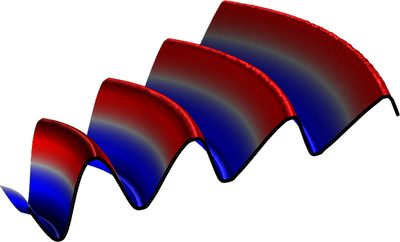# Bibliothèque d'Applications

## Nonlinear Propagation of a Cylindrical Wave — Verification Model

Application ID: 88771

This model example shows how to model nonlinear propagation of a cylindrical wave using the Nonlinear Pressure Acoustics, Time Explicit physics interface available in Acoustics Module of COMSOL Multiphysics. The interface implements the system of nonlinear acoustic equations in the form of a hyperbolic conservation law using the time-explicit discontinuous Galerkin finite element method.

This example analyzes the wave propagation in a lossless media over distances larger that the shock formation distance. Thus the tutorial puts a special emphasis on the techniques necessary for treating solution discontinuities, such as limiters, discretization, and solver settings. The numerical solution is compared to the analytical solution valid at the distances before the shock formation.This model example illustrates applications of this type that would nominally be built using the following products: